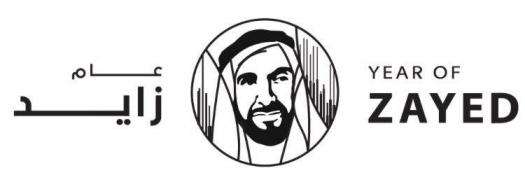# Lesson 10.3: Area Of Triangles

Approved & Edited by ProProfs Editorial Team
At ProProfs Quizzes, our dedicated in-house team of experts takes pride in their work. With a sharp eye for detail, they meticulously review each quiz. This ensures that every quiz, taken by over 100 million users, meets our standards of accuracy, clarity, and engagement.
| Written by Bfemi84
B
Bfemi84
Community Contributor
Quizzes Created: 1 | Total Attempts: 221
Questions: 5 | Attempts: 221SettingsFind the area of triangles

• 1.

### What is the area of a triangle with base 10 cm and height 25 cm?

• A.

35 sq cm

• B.

62.5 sq cm

• C.

125 sq cm

• D.

250 sq cm

C. 125 sq cm
Explanation
The area of a triangle can be calculated using the formula: Area = (base * height) / 2. In this case, the base is given as 10 cm and the height is given as 25 cm. Plugging these values into the formula, we get: Area = (10 * 25) / 2 = 250 / 2 = 125 sq cm.

Rate this question:

• 2.

### What is the area of a parallelogram with base 30 ft and height 25 ft?

• A.

55 sq ft

• B.

187.5 sq ft

• C.

375 sq ft

• D.

750 sq ft

D. 750 sq ft
Explanation
The area of a parallelogram can be calculated by multiplying the base length by the height. In this case, the base is given as 30 ft and the height is given as 25 ft. Therefore, the area of the parallelogram is 30 ft x 25 ft = 750 sq ft.

Rate this question:

• 3.

### Find the area of a triangle with base of 4 in and height of 6 in.

• A.

12 sq in

• B.

24 sq in

• C.

12 in

• D.

24 in

A. 12 sq in
Explanation
To find the area of a triangle, we can use the formula A = (1/2) * base * height. In this case, the base is given as 4 inches and the height is given as 6 inches. Plugging these values into the formula, we get A = (1/2) * 4 * 6 = 12 square inches. Therefore, the correct answer is 12 sq in.

Rate this question:

• 4.

### The base of a triangle with area of 40 cm2 and height of 5 cm is _________.

• A.

400 sq cm

• B.

16 sq cm

• C.

8 sq cm

• D.

20 cm

B. 16 sq cm
Explanation
The area of a triangle is calculated by multiplying the base length by the height and dividing the result by 2. In this case, the area is given as 40 cm2 and the height is given as 5 cm. By rearranging the formula, we can solve for the base length. Multiplying the height by 2 and then dividing the area by the result gives us the base length of 16 cm. Therefore, the correct answer is 16 sq cm.

Rate this question:

• 5.

### The area of a parallelogram is twice the area of congruent triangles formed by the parallelogram

• A.

False

• B.

TrueBack to top